#beanz Magazine

## Floating Point Numbers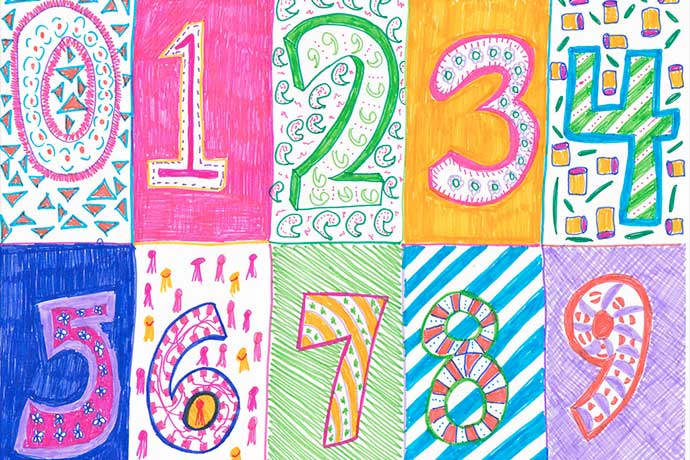Denise Krebs on Flickr

While many people are familiar with floating point numbers in school math problems, they're very useful in computing.

### What Are Floating Point Numbers?

Let’s begin with a definition of floating point numbers. Real numbers are expressed as fixed point numbers or floating point numbers. A fixed point number has a radix point, or decimal point, at a fixed location somewhere in the collection of numbers. For example:

`100.22`

This number represents 100 with two digits or hundredths of value, the two zeroes in this case to the right of the decimal point. We’re used to seeing this sort of fixed point number when we see or write down amounts of money.

With floating point numbers, the decimal point might appear in many possible places in a set of numbers. Floating point numbers contain a collection of numbers, a base number, and an exponent to help determine where to place the decimal point. For example:

`100.22 = 10022 x 10-2`

In this example, our currency value of 100.22 can be represented in a computer as 10022 (called the significand) times 10 (the base number) with -2 as exponent. The relative position of the decimal point is indicated by the exponent. The -2 exponent tells the computer to place the decimal point 2 spaces counting from the right least significant edge of the number, which results in 100.22. If the exponent was a positive number, we would count from the left.

Floating point numbers make it easy to manage very large and small numbers. For example, an astronomer might want to do calculations with the speed measured in seconds of a moon around a planet.

Here’s another example:

`100.220011 = 10022 x 10-6`

In this example, the exponent -6 positions the decimal point six spaces counting from the right edge of the number, or 100.220011. While we have used base 10 in these two examples, computers can use 2 (binary), 10 (decimal), or 16 (hexadecimal) as base numbers. You also could calculate with base 3, in some cases. Base 10 is simplest to demonstrate here.

### Floating Point Numbers and Computers

In computer hardware and software, fixed point numbers can cause problems when they’re computed and stored. Our 100.22 fixed point number, for example, would be translated by a computer into a binary number. The computer also would have to manage the decimal point information when doing calculations with the number. While floating point numbers include the number with a base number and exponent — for example, 10022 x 10-2 — to indicate decimal point location, processing this information takes computer resources.

Every computer has a special chip called an FPU or Floating Point Unit. Computer speed also can be measured in terms of FLOPS, or Floating Point Operations Per Second, the speed the computer can process floating point numbers.

Which leads to an interesting point: there are different ways to calculate floating point numbers. Since the 1990s most computers have used the IEEE 754 standard to ensure computers return the same results when calculating these numbers.

Here’s another interesting point about how computers store and compute numbers: results do not have to be precise. They only have to be reasonable. Programmers who code with floating point numbers, for example, to store and retrieve numbers in a database, must pay close attention to ensure their code does not accidentally change these numbers.

How does this work in the real world? A bank might use a computer to store a transaction for \$100.22 as a fixed number or 10022 x 10-2 as a floating point number, then use code to represent the value as dollars and cents only when displayed in a web page or other software. The computer stores the number in a way that works best for computers while humans see the value they recognize as currency.

There is a lot more to floating point numbers and computing, of course. Follow the links below to discover more details.

#### What can be done to programming languages to avoid floating point pitfalls?

http://programmers.stackexchange.com/questions/62948/what-can-be-done-to-programming-languages-to-avoid-floating-point-pitfalls

#### Floating point arithmetics in 19 programming languages

http://itreallymatters.net/post/386327451/floating-point-arithmetics-in-19-programming

#### Model Monetary Data

This tutorial shows how you handle monetary data and floating point numbers with the MongoDB database. It’s a great practical example of how coders think through the problem.
http://docs.mongodb.org/manual/tutorial/model-monetary-data/

#### Floating Point Operations Per Second (FLOPS)

http://en.wikipedia.org/wiki/FLOPS

#### What Every Computer Scientist Should Know About Floating-Point Arithmetic

http://docs.oracle.com/cd/E19957-01/806-3568/ncg_goldberg.html

### Also In The February 2015 Issue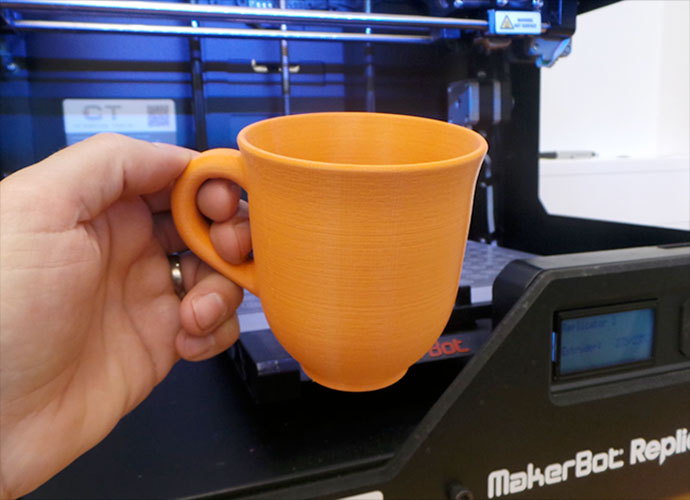### What is 3D Printing?

A basic introduction to 3D printing with concepts, examples, and a glossary of 3D terminology.### Gnomes, Gnomedozers, Spoonriders, and Zen Gnomes

Why are there so many gnomes available for 3D printing?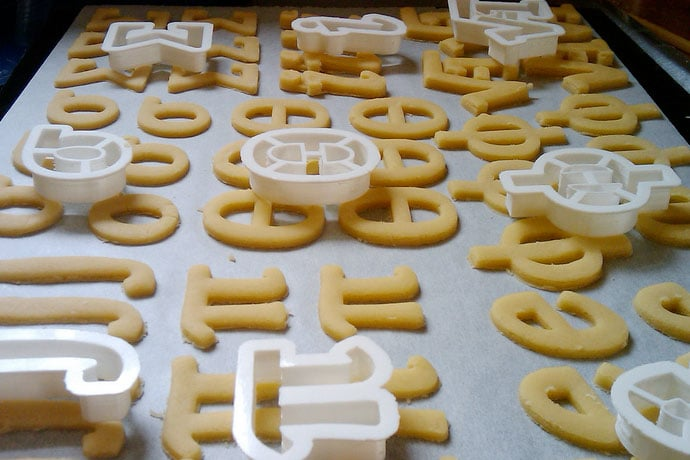### 3D Projects

From simple to complex, nine three-dimensional models you can download then print to explore 3D printing.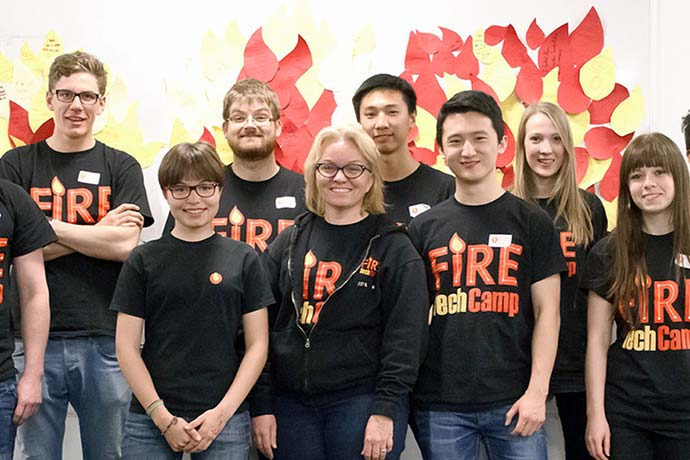### Jill Hodges Talks about Fire Tech Camp

An American living in the United Kingdom, Jill Hodges talks about how she started a summer technology camp for kids.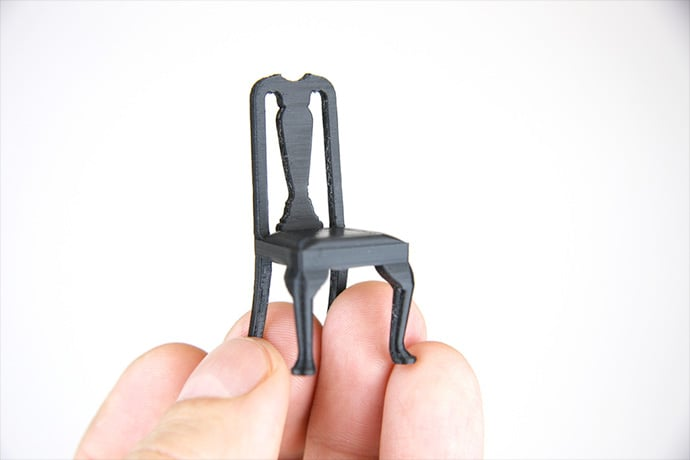### What 3D Models Print Best?

When you create a three-dimensional model for printing, it's important to know what prints well and what does not.### Two Books Teach Coding and Computer Science

One book teaches JavaScript in a fun way, the other computer science as fable.### Lateral Thinking Puzzles

Practice problem solving skills programmers use every day.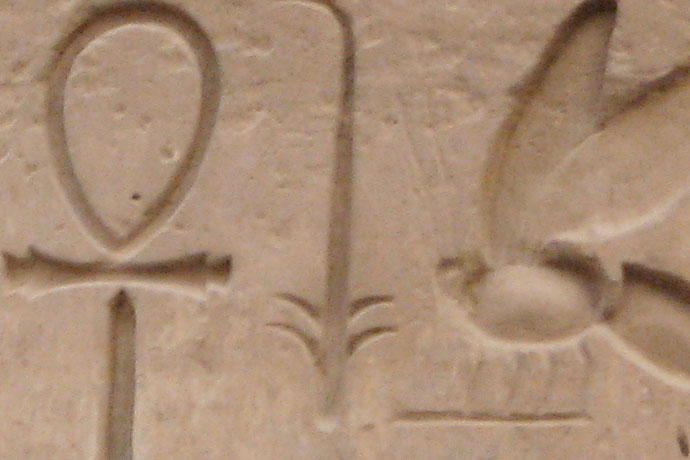Of 8500 plus programming languages, many are forgotten or lightly used enough to be called dead programming languages.### Where Can I Find Local 3D Printing Services?

It's possible to print your 3D project the same way you print a flyer at the UPS store. Here are several options.

### Whatever good things we build end up building us.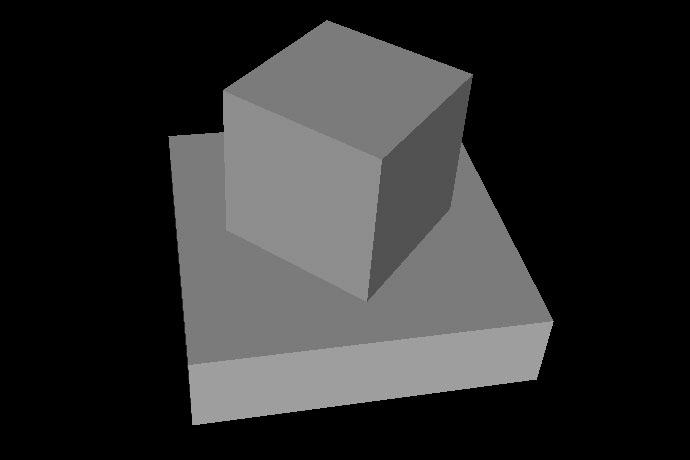### VRML

The VRML language, or Virtual Reality Modeling Language, is ancient but still lets you create simple three-dimensional objects.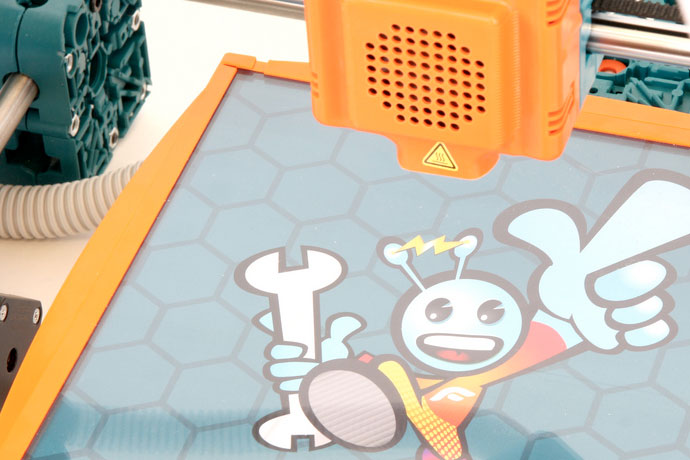### 3D Printers

3D printers may be new technology but there are several printers individuals, schools, and businesses might buy.Links from the bottom of all the February 2015 articles, collected in one place for you to print, share, or bookmark.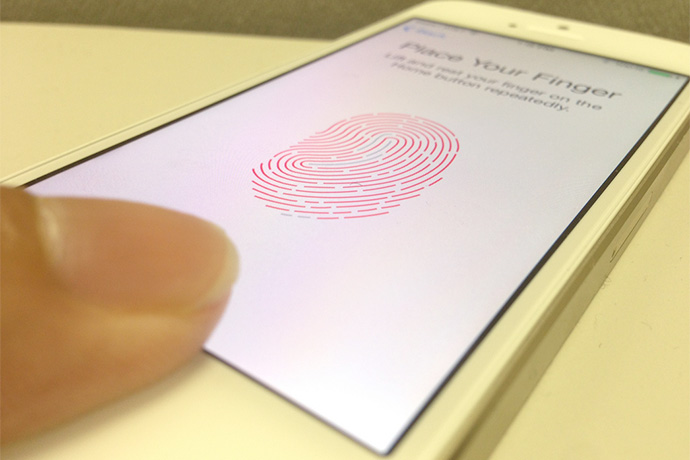### February 2015 News Wire

Interesting stories about computer science, software programming, and technology for December 2014 and January 2015.### Floating Point Numbers

While many people are familiar with floating point numbers in school math problems, they're very useful in computing.